您可以捐助，支持我们的公益事业。 1元 10元 50元 认证码：必填求知 文章 文库 Lib 视频 iPerson 课程 认证 咨询 工具 讲座 Modeler Code要资料订阅捐助
python中利用matplotlib绘图可视化知识归纳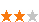263 次浏览 评价： 好 中 差
2020-3-17

 编辑推荐: 本文讲了统计作图函数，为项目设置matplotlib参数，线条相关属性标记设置，颜色设置，修改坐标范围，希望对大家有帮助。 本文来自于cnblogs，由火龙果软件Delores编辑，推荐。

（1）matplotlib图标正常显示中文

import matplotlib.pyplot as plt

plt.rcParams['font.sans-serif']=['simhei'] #用于正常显示中文标签

plt.rcParams['axes.unicode_minus']=False #用于正常显示负号

（2）统计作图函数：

plt.plot()绘制线性二维图，折线图

plt.bar() 绘制条形图

plt.scatter() 绘制散点图

plt.hist() 绘制二维条形直方图，显示数据的分配情况

plt.pie() 绘制饼图

plt.boxplot() 绘制箱形图

（3）为项目设置matplotlib参数

figure: 控制dpi、边界颜色、图形大小、和子区( subplot)设置

font: 字体集（font family）、字体大小和样式设置

grid: 设置网格颜色和线性

legend: 设置图例和其中的文本的显示

line: 设置线条（颜色、线型、宽度等）和标记

xticks和yticks: 为x,y轴的主刻度和次刻度设置颜色、大小、方向，以及标签大小。

axex: 设置坐标轴边界和表面的颜色、坐标刻度值大小和网格的显示

backend: 设置目标暑促TkAgg和GTKAgg

patch: 是填充2D空间的图形对象，如多边形和圆。控制线宽、颜色和抗锯齿设置等。

savefig: 可以对保存的图形进行单独设置。例如，设置渲染的文件的背景为白色。

（4）线条相关属性标记设置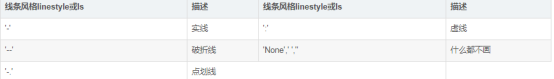marker：对坐标点标记（线条标记）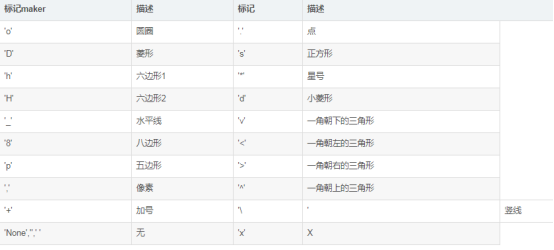（5）颜色设置

（6）修改坐标范围

plt.axis(xmin,xmax,ymin,ymax) 给定了坐标范围

xlim(xmin,xmax)和ylim(ymin,ymax)来调整x,y的坐标范围

（7）设置轴标记plt.xticks()/plt.yticks()：人为设置坐标轴的刻度显示的值

import matplotlib.pyplot as plt

import numpy as np

plt.figure(figsize=(8,6), dpi=80) # 创建一个新的 1 * 1 的子图，接下来的图样绘制在其中的第 1 块（也是唯一的一块）

plt.subplot(1,1,1)

X = np.linspace(-np.pi, np.pi, 256,endpoint=True)

C,S = np.cos(X), np.sin(X)

plt.plot(X, C, color="blue", linewidth=1.0, linestyle="-") # 绘制余弦曲线，使用蓝色的、连续的、宽度为 1 （像素）的线条

plt.plot(X, S, color="r", lw=4.0, linestyle="-") # 绘制正弦曲线，使用绿色的、连续的、宽度为 1 （像素）的线条

plt.axis([-4,4,-1.2,1.2]) #给定坐标轴的范围

# 设置轴记号

plt.xticks([-np.pi, -np.pi/2, 0, np.pi/2, np.pi],

[r'$-\pi$', r'$-\pi/2$', r'$0$', r'$+\pi/2$', r'$+\pi$'])

plt.yticks([-1, 0, +1],

[r'$-1$', r'$0$', r'$+1$']) #注意：这里设置记号时，同时设置了记号的标签。使用了LaTeX

plt.show()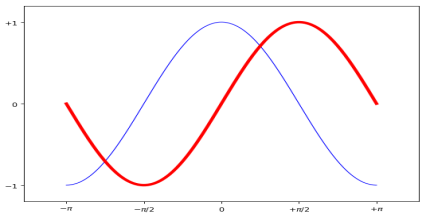（8）叠加图：用一条指令画出多条不同格式的线

import matplotlib.pyplot as plt

import numpy as np

t = np.arange(0., 5., 0.2)

plt.plot(t, t, 'r--', t, t**2, 'bs', t, t**3, 'g^')

plt.show()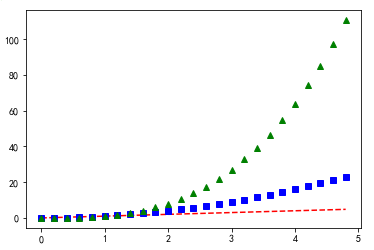（9）创建子图plt.subplot()

import matplotlib.pyplot as plt

plt.figure(1) # 第一张图

plt.subplot(211) # 第一张图中的第一张子图

plt.plot([1,2,3])

plt.subplot(212) # 第一张图中的第二张子图

plt.plot([4,5,6])

plt.figure(2) # 第二张图

plt.plot([4,5,6]) # 默认创建子图subplot(111)

plt.figure(1) # 切换到figure 1 ; 子图subplot(212)仍旧是当前图

plt.subplot(211) # 令子图subplot(211)成为figure1的当前图

plt.title('Easy as 1,2,3') # 添加subplot 211 的标题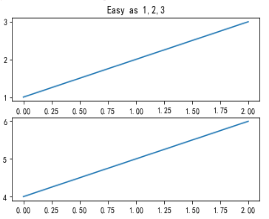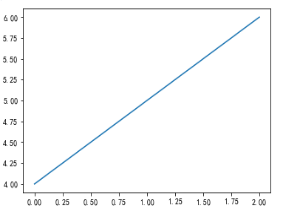（10）plt.text()添加文字说明

Text语法说明：

text(x,y,string,fontsize=15,verticalalignment="top",horizontalalignment="right")

x,y:表示坐标值上的值

string:表示说明文字

fontsize:表示字体大小

verticalalignment：垂直对齐方式 ，参数：[ ‘center’ | ‘top’ | ‘bottom’ | ‘baseline’ ]

horizontalalignment：水平对齐方式 ，参数：[ ‘center’ | ‘right’ | ‘left’ ]

bbox给标题增加外框 其中常用参数： boxstyle方框外形，facecolor(简写fc)背景颜色，edgecolor(简写ec)边框线条颜色，edgewidth边框线条大小

import matplotlib.pyplot as plt

plt.text(0.6, 0.5, "test", size=50, rotation=30.,ha="center", va="center",bbox=dict(boxstyle="round",ec=(1., 0.5, 0.5),fc=(1., 0.8, 0.8),))

plt.text(0.5, 0.4, "test", size=50, rotation=-30.,ha="right", va="top",bbox=dict(boxstyle="square",ec=(1., 0.5, 0.5),fc=(1., 0.8, 0.8),))

plt.draw()

plt.show()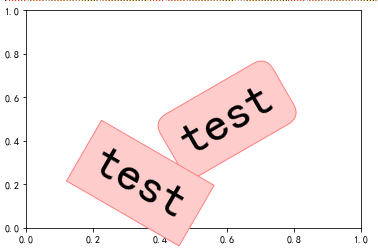（12）文本注释annotate()

annotate()参数设置箭头指示的位置，xytext参数设置注释文字的位置

---arrowprops参数以字典的形式设置箭头的样式

import numpy as np

import matplotlib.pyplot as plt

plt.figure(figsize=(6, 6))

x = np.random.randint(0, 10, size=10)

x = 30 # 对x中索引值为5的重新赋值

plt.plot(x)

plt.ylim([-2, 35])

# plt.annotate(s="this point is important", xy=(5, 30), xytext=(6, 31),arrowprops={"width": 2, "headlength": 5, "headwidth": #5, "shrink": 0.1})

plt.annotate(s="this point is important", xy=(5, 30), xytext=(6, 31),arrowprops={"arrowstyle":"->"}) # 如果arrowprops中有arrowstyle,就不应该有其他的属性，xy代表的是箭头的位置，xytext代表的是箭头文本的位置。

plt.show()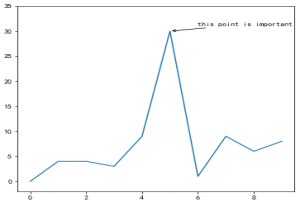（13）plt.title()设置图像标题

title常用参数:fontsize设置字体大小、fontweight设置字体粗细、fontstyle设置字体类型、verticalalignment设置水平对齐方式 ，可选数： ： 'center' , 'top' , 'bottom' ,'baseline'

horizontalalignment设置垂直对齐方式，可选参数：left,right,center

rotation(旋转角度)可选参数为:vertical,horizontal 也可以为数字

alpha透明度，参数值0至1之间

backgroundcolor标题背景颜色

（14）Matplotlib中的fill_between()函数总结

l 示例：曲线覆盖

import numpy as np

import matplotlib.pyplot as plt

x = np.array([i for i in range(30)])

y = np.random.rand(30)

plt.plot(x, y)

plt.fill_between(x, 0, y, facecolor='green', alpha=0.3)

plt.show()

x：第一个参数表示覆盖的区域，直接复制为x，表示整个x都覆盖

0：表示覆盖的下限

y： 表示覆盖的上限是y这个曲线

facecolor:覆盖区域的颜色

alpha：覆盖区域的透明度[0,1]，其值越大，表示越不透明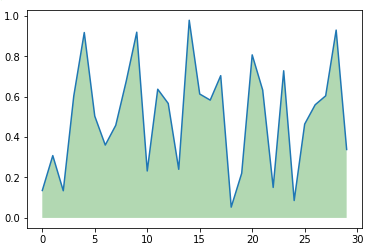y1 = np.random.rand(30)

y2 = y1 + 0.3

plt.plot(x, y1,'b',x,y2,'r')

plt.fill_between(x, y1, y2, facecolor='green', alpha=0.3)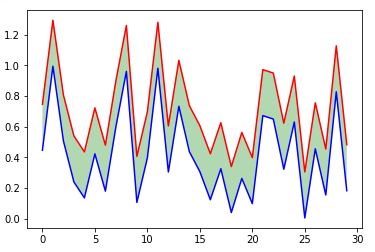import numpy as np

import matplotlib.pyplot as plt

x = np.array([i for i in range(30)])

print(x)

y = np.random.rand(30)

# 设置想要高亮数据的位置

position = [[1, 6],

[10, 12],

[20, 23],

[26, 28]]

print(position)

# 画图

plt.plot(x, y, 'r')

for i in position:

print(i) #输出结果i为：[1, 6], [10, 12], [20, 23], [26, 28]

print(x[i:i]) #结果为：[1 2 3 4 5] [10 11] [20 21 22] [26 27]

plt.fill_between(x[ i : i ], 0, 1, facecolor='green', alpha=0.3)

plt.show()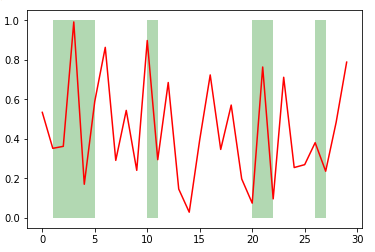263 次浏览     评价： 好 中 差订阅捐助
 相关文章 手机软件测试用例设计实践 手机客户端UI测试分析 iPhone消息推送机制实现与探讨 Android手机开发（一）
 相关文档 Android_UI官方设计教程 手机开发平台介绍 android拍照及上传功能 Android讲义智能手机开发
 相关课程 Android高级移动应用程序 Android系统开发 Android应用开发 手机软件测试